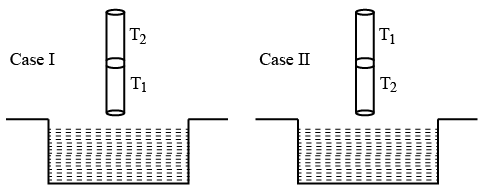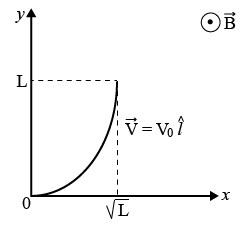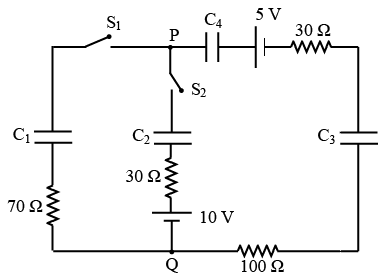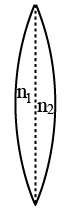Instructions

For the following questions answer them individually

Question 1

# Consider a spherical gaseous cloud of mass density $$\rho$$(r) in free space where r is the radial distance from its center. The gaseous cloud is made ofparticles of equal mass m moving in circular orbits about the common center with the same kinetic energy K. The force acting on the particles is their mutual gravitational force. If $$\rho$$(r) is constant in time, the particle numberdensity n(r) = $$\rho$$(r)/m is[G is universal gravitational constant]Question 2

# A thin spherical insulating shell of radius R carries a uniformly distributed charge such that the potential at its surface is $$V_0$$. A hole with a small area $$\alpha 4 \pi R^2 (\alpha \ll 1)$$ is made on the shell without affecting the rest of the shell. Which one of the following statements is correct?Question 3

# A current carrying wire heats a metal rod. The wire provides a constant power (P) to the rod. The metal rod is enclosed in an insulated container. It is observed that the temperature (T) inthe metal rod changes with time (t) as$$T(t) = T_0 (1 + \beta t^{\frac{1}{4}}),$$where $$\beta$$ is a constant with appropriate dimension while $$T_0$$ is a constant with dimension of temperature. The heat capacity of the metal is,Question 4

# In a radioactive sample, $$_{19}^{40}K$$ nuclei either decay into stable $$_{20}^{40}Ca$$ nuclei with decay constant $$4.5 \times 10^{-19}$$ per year or into stable $$_{18}^{40}Ar$$ nuclei with decay constant $$0.5 \times 10^{-10}$$ per year. Given that in this sample all the stable $$_{20}^{40}Ca$$ and $$_{18}^{40}Ar$$ nuclei are produced by the $$_{19}^{40}K$$ nuclei only. In time $$t \times 10^9$$ years, if the ratio of the sum of stable $$_{20}^{40}Ca$$ and $$_{18}^{40}Ar$$ nuclei to the radioactive $$_{19}^{40}K$$ nuclei is 99, the value of t will be,[Given: $$\ln 10 = 2.3$$]Question 5

# A cylindrical capillary tube of 0.2 mm radius is made by joining two capillaries T1 and T2 of different materials having water contact angles of $$0^\circ$$ and $$60^\circ$$, respectively. The capillary tube is dipped vertically in water in two different configurations, case I and II as shown in figure. Which of the following option(s) is(are) correct? [Surface tension of water = 0.075 N/m, density of water = $$1000 kg/m^3$$, take $$g = 10 m/s^2$$]Question 6

# A conducting wire of parabolic shape, initially $$y = x^2$$, is moving with velocity $$\overrightarrow{V} = V_0 \widehat{l}$$ in a non-uniform magnetic field $$\overrightarrow{B} = B_0 \left( 1 + \left(\frac {y}{L}\right)^\beta \right) \widehat{k}$$ as shown in figure. If $$V_0, B_0, L$$ and $$\beta$$ are positive constants and $$\triangle\phi$$ is the potential difference developed between the ends of the wire, then the correct statement(s) is/are:Question 7

# In the circuit shown,initially there is no charge on capacitors and keys $$S_1 and S_2$$ are open. The values of the capacitors are $$C_1 = 10 \mu F, C_2 = 30 \mu F and C_3 = C_4 = 80 \mu F.$$Which of the statement(s) is/are correct?Question 8

# A charged shell of radius R carries a total charge Q. Given $$\phi$$ as the flux of electric field through a closed cylindrical surface of height h, radius r and with its center same as that of the shell. Here, center of the cylinder is a point on the axis of the cylinder which is equidistant from its top and bottom surfaces. Which of the following option(s) is/are correct?[$$\epsilon_0$$ is the permittivity of free space]Question 9

# One mole of a monatomic ideal gas goes through a thermodynamic cycle, as shown in the volume versus temperature (V-7 ) diagram. The correct statement(s) is/are:[R is the gas constant]Question 10

# A thin convex lens is made of two materials with refractive indices $$n_1 and n_2$$ as shown in figure. The radius of curvature of the left and right spherical surfaces are equal. f is the focal length of the lens when $$n_1 = n_2 = n.$$ The focal length is $$f + \triangle f$$ when $$n_1 = n$$ and $$n_2 = n + \triangle n$$. Assuming $$\triangle n \ll (n — 1)$$ and 1 < n < 2, the correct statement(s) is/areOR